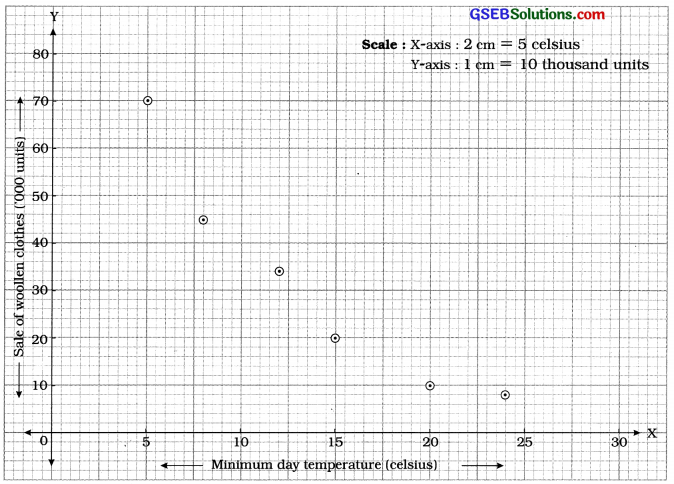# GSEB Solutions Class 12 Statistics Part 1 Chapter 2 Linear Correlation Ex 2.1

Gujarat Board Statistics Class 12 GSEB Solutions Part 1 Chapter 2 Linear Correlation Ex 2.1 Textbook Exercise Questions and Answers.

## Gujarat Board Textbook Solutions Class 12 Statistics Part 1 Chapter 2 Linear Correlation Ex 2.1

Question 1.
A ball pen making company wants to know the relation between the price (in ₹) and supply (in thousand units) of its most selling Gel Pen. The following information is collected for it. Draw a scatter diagram and interpret it.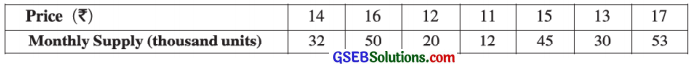X = Price (in ₹), Y = Monthly Supply (‘000 units).
Plotting the points corresponding to the ordered pairs (14, 32), (16, 50), (12, 20), (11, 12), (15, 45), (13, 30) and (17, 53) of variables X and Y on the graph paper the scatter is obtained as follows: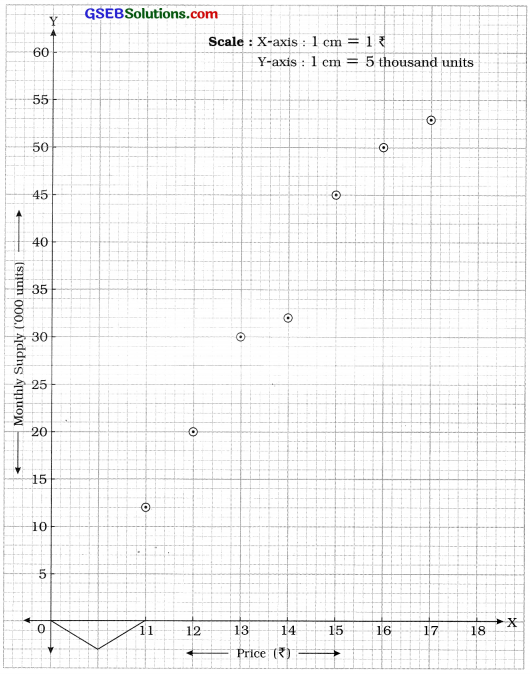Interpretation: It is clear from the scatter diagram that all the points do not lie on the same line. Here, the changes in price and supply are in the same direction but not in the same proportion. Hence, all the points are not on the same line. So, there is partial positive correlation price and supply.Question 2.
A Company manufactures R.O. plants for the factories. The information about the advertisement cost for its sale and the profit from the sale of R.O. plants is given below: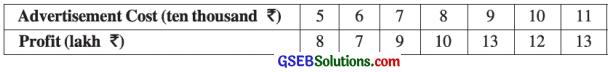Draw a scatter diagram from this information and state the nature of the relationship between the advertisement cost and profit earned from the sale of R.O. plants.
X = Advertisement cost (‘0000 ₹) and Y = Profit (in lakh ₹).
Plotting the points corresponding to ordered pairs (5, 8), (6, 7), (7, 9), (8, 10), (9, 13), (10, 12), (11, 13) of variables X and Y on the graph paper, the scatter diagram is obtained as follows: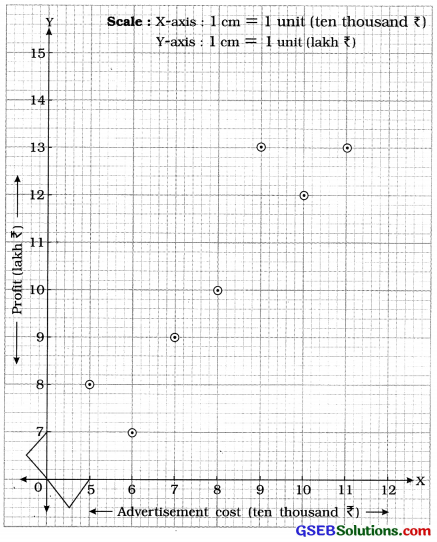Interpretation: It is clear from the scatter diagram that all the points do not lie on the same line. Here, the changes in advertisement cost and profit are in the same direction but not in the same proportion. Hence, all the points are not on the same line. So, there is partial positive correlation between advertisement cost and profit.Question 3.
The following information is collected to study the relationship between the minimum day temperature and sale of woollen clothes during a particular day of winter for six different cities: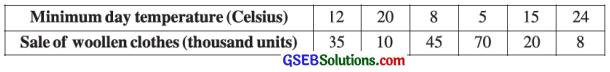Draw a scatter diagram from this information and interpret it.